The Concave mirror - u-v method

# Objective

To find the focal length of the given concave mirror by

(i)  by u-v method

(ii) from u - v graph

(iii) from   1/u - 1/(v ) graph

# Theory

## Spherical mirrors

It is a mirror which has the shape of a piece cut out of a spherical surface. Two types of spherical mirrors are;

• Concave mirror: Its inner concave surface reflects, and has polished outer surface.
• Convex mirror: Its outer convex surface reflects, and has polished inner surface.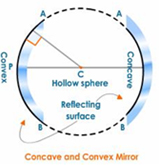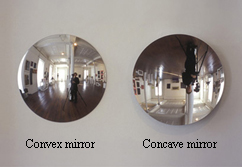Different terms associated with spherical mirrors are;

Pole (P): The centre of the spherical mirror.

Centre of curvature (C): The centre of the sphere, of which the mirror is a part.

Principal focus (F): The point on the principal axis, on which all parallel rays meet after reflection.

Radius of curvature (R): The distance between pole and centre of curvature.

Focal length (f): The distance between pole and principal focus.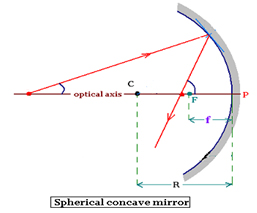## Concave Mirror

Concave mirrors have the reflecting surface that bulges inward. They are also called converging mirrors because it converges all parallel beam of light incident on it. Unlike a flat mirror, concave mirrors can form real images that are projected out in front of the mirror at the place where the light focuses. Concave mirrors can be used in satellite dishes, vehicle headlights, astronomical telescopes and many more areas.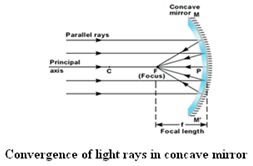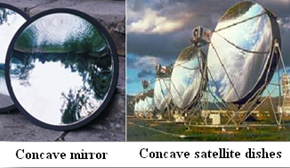## Mirror Formula

The equation connecting the distance between mirror and object (u), distance between mirror and image (v), and the focal length of the mirror (f) is called mirror formula.Or ; The focal length of the concave mirror,Focal length by graphical method

From u-v graph :

We can measure the focal length of the given concave mirror graphically by plotting graph between u and v. For this, plot a graph with u along X axis and v along Y axis by taking same scale for drawing the X and Y axes. A curve is obtained. The point at which the bisector meets the curve gives the radius of curvature (R).

Now focal length can be calculated from the relation, R = 2f.

From 1/u – 1/v graph :

We can also measure the focal length by plotting graph between 1/-u and 1/v. Plot a graph with 1/u along X axis and 1/v along Y axis by taking same scale for drawing the X and Y axes. The graph is a straight line intercepting the axes at A and B.

The focal length can be calculated by using the relations, OA=OB= 1/f.

# Learning Outcomes

• Students understand different types of mirrors and their image properties.
• Students learn and apply the mirror formula.
• Students understand the different terms associated with spherical mirrors.
• Students recall the applications of concave mirror.

Cite this Simulator: